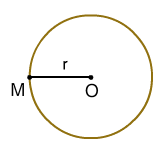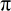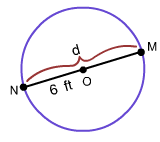Print Rate 0 stars
Lesson size:
Message preview:
Someone you know has shared lesson with you:

To play this lesson, click on the link below:

Hope you have a good experience with this site and recommend to your friends too.

Login to rate activities and track progress.
Login to rate activities and track progress.

The radius of a circle is a line segment that goes from the center point to a point on the circle. Thus, it goes halfway through the circle.## Diameter

The diameter of circle is a line segment that goes all the way across a circle through the center point.## Circumference

The circumference of a circle is the distance around the outside of the circle.The radius, diameter, and circumference of a circle always have the same relationships. Thus, if you know one of these, you can calculate the other two.

The diameter is always twice as long as the radius. This makes sense because the diameter is all the way across the circle while the radius is only halfway across.When circumference is involved, the relationship is a little bit more complex because of the number(Pi).

### Did you know that if you divide the circumference of any circle by its diameter, you will always get the same exact number every time?

The number you get is called(Pi) and is approximately equal to 3.14, but the decimal places actually keep going and going.

Computers have calculated millions and even billions of digits to the number, but they haven't been able to find the end of it yet.

When you usein a problem, typically you can just rememberis approximately 3.14.

There are three formulas you can use when dealing with the circumference:

In these formulas, C = circumference, r = radius, and d = diameter

1) Use the formula given below to find the circumference when you know the radius.

C = 2xxr

2) Use the formula given below to find the circumference when you know the diameter.

C =xd

3) Use the formula given below to find the diameter when you know the circumference.

C = c ÷Let's take a look at some examples:

### Example 1

Find the radius, diameter, and circumference of each circle.Radius = 4 inches (Remember the radius goes halfway across the circle.)

The diameter is always twice as long as the radius.

So, the diameter will be:

4 x 2 = 8

The diameter is 8 inches.

For the circumference, we need to choose a formula.

It's usually better to use the information we are given (instead of the information we figured out ourselves - just in case we made a mistake).

We started with r = 4. The first formula C = 2xxr has r in it, so we will start with that formula and substitute in the information we know.

C = 2xxr

This formula says we must multiply 2 times Pi (3.14) times the radius

C = 2x 3.14 xr

Then we just need to multiply:

C = 25.12 in.

Answer: Radius = 4 in., diameter = 8 in., circumference =25.12 in.Diameter = 6 feet (as shown in the above picture)

The diameter is always twice as long as the radius.

So, if our diameter is 6 feet, the radius must be half as long as that.

6 ÷ 2 = 3

So the radius is 3 feet long.

For the circumference, we need to choose a formula. Remember it's usually better to use the information we are originally given. We started with d = 6.

The first formula C =xd has d in it, so we will start with that formula and substitute in the information we know.

C =xd

This formula says we must multiply Pi (3.14) by the diameter.

C = 3.14x6

Then we just need to multiply:

C = 18.84 ft.

Diameter = 6 ft.,

Circumference = 18.84 ft.We know that the circumference = 6.28 meters because we are told this, but we need to find the diameter or radius.

The formula given below should help us do that:

d = c ÷This formula says that to find the diameter, we must take the circumference and divide it by Pi (3.14)

d = 6.28 ÷ 3.14

On simplifying we get :

d = 2 meters

Now, as we know the diameter, it is easy to find the radius. The diameter is twice as long as the radius, so the radius is half as long as the diameter.

2 ÷ 2 = 1

So, the radius is 1 meter long.

(And this answer makes sense. If halfway across the circle is 1 meter, all the way across is 2 meters).

Answer: C = 6.28 m., d ? 2 m., r ? 1 m.

Parts of circle

A line segment that goes from the center point to a point on the circle. Thus, it goes halfway through the circle.2. Diameter
A line segment that goes all the way across a circle through the center point.3. Circumference
The distance around the outside of the circle.The diameter is always twice as long as the radius ; d = 2 x r.

Important formulas: C = 2xxr , C =xd , d = C ÷(Pi) is a special number that occurs in circles. Its decimal places keep going and going, but we can use the approximation 3.14. So, in calculations, think? 3.14.

## Similar Lessons

Become premium member to get unlimited access.